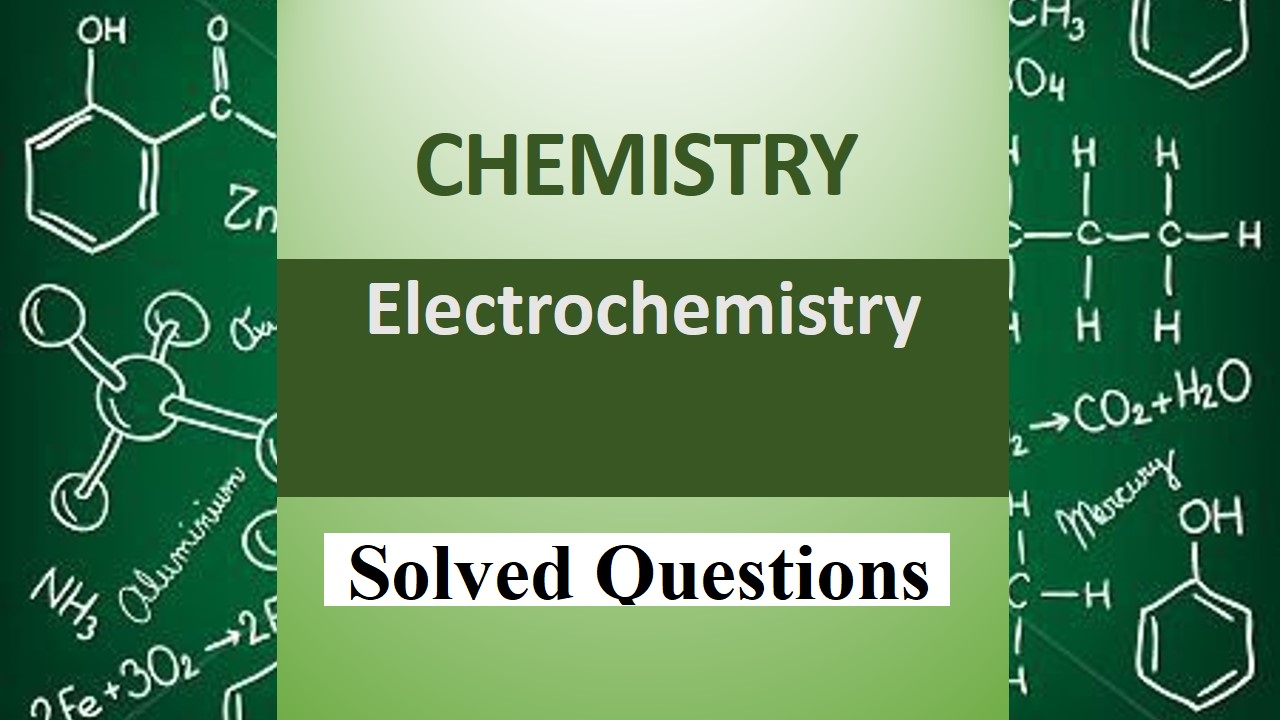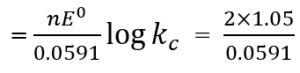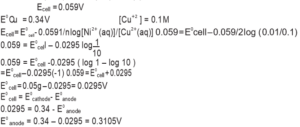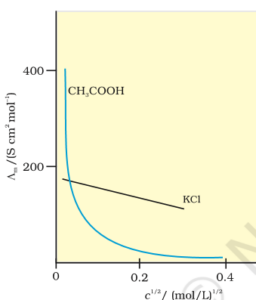# Solved Questions Electrochemistry## Solved Questions Electrochemistry

Solved Questions Electrochemistry:

Que 1. How much electricity in terms of Faraday is required to produce 100g of Ca from molten CaCl2?

Ans 1.  5F

Que 2. Name the solid substance produced, during the discharge of lead- storage battery.

Que 3. If 0.5 ampere current flows through a wire for 2 hours. Calculate the number of electrons through the wire.

Ans 3.         I = 0.5 A

t = 2 hours = 2 × 60 × 60 s = 7200 s

Q = It = 0.5 A × 7200 s = 3600 C

96500 C is equivalent of 1 mole = 6.02 × 1023 electrons

3600 C is equivalent of 1mole = 6.02 × 1023 × 3600/96500

= 2.246 × 1022 electrons.

Que 4. Write the importance of salt bridge in a Galvanic cell?

Ans 4. i). It completes the cell circuit by connecting the solutions of two half cells.

ii). it maintains the electrical neutrality of the two solutions in the two half cells.

Que 5. What is a fuel cell?  Write two chemicals that can be used as fuel.

Ans 5. The cell that converts the combustion energy of fuel into electricity. Methane and hydrogen can be used as fuel.

2H2(g) + 4OH(aq) → 4H2O(l) + 4e

O2 + 2H2O (l) + 4e → 4OH(aq).

Que 6. How many moles of mercury will be produced by electrolysing 1.0 M Hg(NO3)2 solution with a current of 2.00 A for three hours? Molar mass of Hg(NO3)2 = 200.6 g/mol

Ans 6. Current = 2A   Time = 3h = 3 × 60 × 60s

W = ZIt

Z for Hg in compound = 200.6/2F

W = 200.6 × 2 × 3 × 60 × 60/2(96500)

= 22.45g

= number of moles = 22.45/200.6 = 0.112 mol

Que 7. Represent the galvanic cell in which the following reactions take place

Zn(s) + 2 Ag+(aq) → Zn2+(aq) + 2 Ag(s)

(i) Which one of the electrodes is negatively charged?

(ii) Write the reaction taking place at each of the electrodes.

(iii) Name the carrier of current within the cell.

Ans 7. i) Zn electrode is negatively charged

ii) At anode Zn(s) → Zn2++ 2e

At cathode Ag+(aq) + e → Ag(s)

iii) Ions are the carrier of current within the cell.

Que 8. State Faraday’s laws. How much charge is required for the reduction of 1 mole of Cu2+ to Cu?

Ans 8. Statement Cu2++2e- → Cu

Charge required for the reduction of 1 mole Cu2+ = 2F

= 2 (96500 C) = 193000C

Que 9. What is the role of ZnCl2 in a dry cell?

Ans 9. Zncl2 combines with NH3 to forms a complex salt otherwise due to the pressure of NH3 cell may crack.

Que 10. Write two advantages of H2-O2 fuel cell.

Ans 10. i). Do not cause any type of pollution

ii). They have high efficiency (60% – 70%).

Solved Questions Electrochemistry

Que 11. Determine the value of equilibrium constant and ΔG0 for the following reaction Ni(s) + 2 Ag+(aq) → Ni2+ (aq) + 2 Ag(s) (E0 = 1.05V)

Ans 11.  n = 2

So, ΔG° = -nFE0

= – 2 × 96500 × 1.05

= – 202650 Jmol-1 = -202.65 kJ mol-1log Kc = 35.533

Kc = 3.412 × 1035

Que 12. Calculate the standard electrode potential of Ni2+// Ni electrode if emf of the Ni2+/Ni(0.01) // Cu2+(0.1) / Cu (s)  electrochemical cell is 0.059V. Given E0 = 0.34V.

Ans 12.Que 13. Why does a Galvanic cell become dead after some time?

Ans. 13. As the reaction in the cell precedes the concentration of ions in one half cell increases while in another half-cell decreases hence electrode potential also changes. When concentration becomes equal the E.M.F becomes zero.

Que 14. The following is a plot of molar conductivity of electrolytes A and B against the square root of concentration(i) Write the mathematical relationship between Am and C for strong electrolytes (A)

(ii) Identify the curves represented by a strong electrolyte (A) and weak electrolyte (B)

(iii) What does m represent? Give one method for determining m of the electrolyte (B)

Ans 14: i). Λm = λ0m – A√c

ii). A straight and B curved

iii). To determine the value of limiting molar conductivity for electrolyte B, an indirect method based upon Kohlrausch law of independent migration of ions is used.

Que 15. The conductivity of 0.20 m solution of KCl at 298 K is 0.02485 cm-1. Calculate its molar conductivity.

Ans 15.   λm = ƙ x 1000/molarities

= 0.0248×1000/0.20

= 124.5 cm2mole

Que 16. The resistance of conductivity cell filled with 0.1 M KCl solution is 100 Ohm. If the resistance of the same cell, when filled with 0.02 M KCl solution, is 520 ohm. Calculate the conductivity and molar conductivity of 0.02 M KCl solution. The conductivity of 0.1 M KCl solution is 1.29 m–1.

Ans 16. i) Cell constant G = conductivity (resistance) = 1.29 S m–1 (100Ω) 129 m–1 or 1.29 cm–1

1. ii) Conductivity of 0.02 M KCl solution (k) = cell constant /resistance

=  = 2.48 × 10–3 cm-1

iii) Molar conductivity = k × 1000/M = 2.48 × 10–3 × 1000/0.02 = 124 S cm2 mol

Que 17. λm for NaCl, HCl & Na Ac are 126.4, 425.9 & 91.05 cm2 mol-1 respectively. Calculate λom for HAc.

Ans 17. λom (H Ac) =     λom ( HCl )   +     λom (NaAc)  –   λom(NaCl)

= 425.9 + 91 – 126.4

= 390.55 cmmol-1

Que 18.  The Eo cell for Daniel cell is 1.1 V. Calculate the standard Gibbs energy for the reaction: Zn (s) + Cu2+ (aq)  →   Zn2+ (aq) + Cu (s)

Ans 18. ∆G0 = -n F Eo cell (n=2)

= -2 × 1.1v × 96500 C mol-1

= -212300 J mol-1 = -21.230 KJ/mol

Que19. Account for the following:

(i) Alkaline medium inhibits the rusting.

(ii) Iron does not rust even if zinc coating is broken down at any point in a galvanized pipe.

Ans 19. i) This is due to the alkalinity prevent the availability of H+ ions.

ii). In GI pipes Zinc coating acts as anode & exposed iron acts as a cathode. If zinc undergoes corrosion protecting iron from rusting.

Que 20. i) Define the molar conductivity of a solution and explain how molar conductivity changes with a change in concentration of solution for a weak and strong electrolyte.

ii). The resistance of a conductivity cell containing 0.001M KCl solution at 298K is 1500Ω. What is the cell constant, if the conductivity of 0.01M KCl solution at 298 K is 0.146 × (10–3) S cm–1?

Ans 20. i) Molar conductivity – It is defined as the conductance of the solution which contains one mole of an electrolyte such that the entire solution is in between two electrodes kept one centimeter apart sharing a unit area of cross-section.

(ii) Given conductivity K = 0.146 × (10–3) S cm–1

Cell constant G = k × R  = 0.146 × 10–3 × 1500= 0.219 cm–1

Solved Questions Electrochemistry